# Finite differences: application to PDEs¶

Here's a simple Forward-Euler finite-difference simulation of the 1d heat equation, \begin{equation*} u_t = \nu u_{xx} \end{equation*} on the domain $x \in [0,L_x]$ with Dirichlet boundary conditions $u(0) = u(L_x) = 0$. Here $u(x,t)$ represents the temperature distribution over the spatial variable $x$, evolving over time $t$. The constant $\nu$ (Greek "nu") is the thermal diffusivity of the material. The subscripts are short-hand for differentiation, e.g. $u_t = \partial u /\partial t$.

We discretize space by breaking $x$ into a discrete set of uniformly spaced gridpoints $x_j = j \Delta x$, $j=0,1,\ldots,N_x,N_{x+1}$, where $\Delta x = L_x/(N+1)$. Time is broken into discrete time steps at intervals $\Delta t$. We then discretize the continuous function $u(x,t)$ in space and time as \begin{equation*} u^n_j = u(x_j, n \Delta t) \end{equation*} that is, by considering its value on the gridpoints at the discrete time steps. The vector \begin{equation*} u^n = \left(u_1^n, u_2^n, \ldots, u_{N_x}^n\right) \end{equation*} then represents the function $u(x,t)$ on the interior gridpoints at time $t=n\Delta t$. We don't include $u_0^n$ and $u_{N+1}^n$ in the vector $u^n$ because the boundary conditions $u(0) = u(L_x) = 0$ ensure that these values are always zero.

In class we derived the forward-Euler timestepping update rule that maps the temperature distribution $u^n$ into $u^{n+1}$, its value at the next time step, as a matrix-vector multiplication \begin{equation*} u^{n+1} = \left( I + \nu \frac{\Delta t}{\Delta x^2} D_2 \right)u^n \end{equation*} where $D_2$ is a matrix with -2's on its diagonal and 1's on its sub- and superdiagonals.

The following Julia code sets up an initial condition $u(x) = 10 x^4 (L_x-x)$ and integrates it in time using this Forward-Euler timestepping rule. That particular $u(x)$ was chosen to match the boundary conditions and produce a single spot of concentrated heat, near $x=0.8$.

In :
# Forward-Euler time integration of 1d heat eqn uₜ = ν uₓₓ
Lx = 1      # spatial domain is [0, Lx]
ν  = 1/8    # diffusion constant
T  = 1/2    # total integration time

Nx = 32    # number of gridpoints
Δt = 1/256 # time step

Nt = round(Int, T/Δt)  # total number of timesteps
Δx = Lx/(Nx+1)   # grid spacing
x = Δx*(1:Nx)    # interior gridpoints
t = Δt*(0:Nt-1)  # time steps

# set up a matrix to hold u(x,t) and assign an initial condition
# that fits the boundary conditions u(0) = u(L) = 0.
U  = zeros(Nx,Nt)
U[:,1] = 10*(x.^4).*(Lx-x)

# Set up Forward Euler time-stepping formula
# u^{n+1} = (I + ν Δt/Δx^2 D₂) u^n
# u^{n+1} = L u^n
D₂ = diagm(-2*ones(Nx)) + diagm(ones(Nx-1), -1) + diagm(ones(Nx-1),1)
L  = I + (ν * Δt/Δx^2) * D₂

# iterate Forward Euler time stepping formula
for n = 2:Nt
U[:,n] = L*U[:,n-1]
end

using PyPlot
pcolor(x,t,U',vmin=0, vmax=1)
colorbar()
xlabel("x")
ylabel("t")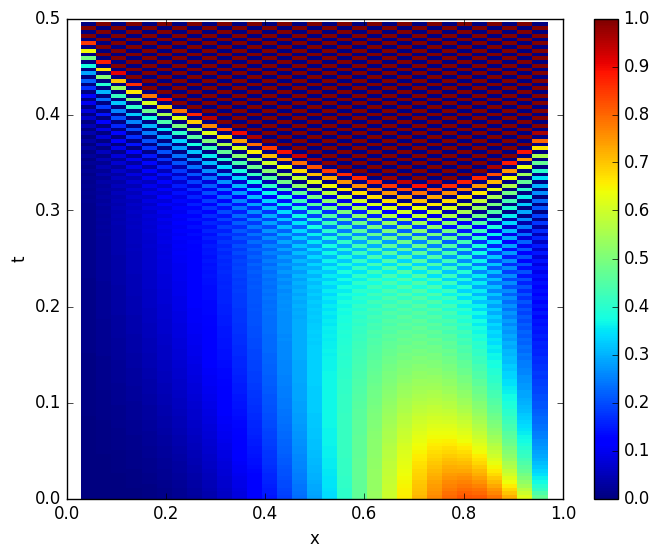Out:
PyObject <matplotlib.text.Text object at 0x7fab1aac7a50>
In :
plot(U[:,1], "b.-")
plot(U[:,round(Int, 0.1/Δt)], "r.-")
plot(U[:,round(Int, 0.2/Δt)], "m.-")
plot(U[:,round(Int, 0.3/Δt)], "g.-")

xlabel("x")
ylabel("u(x,t)")
grid("on")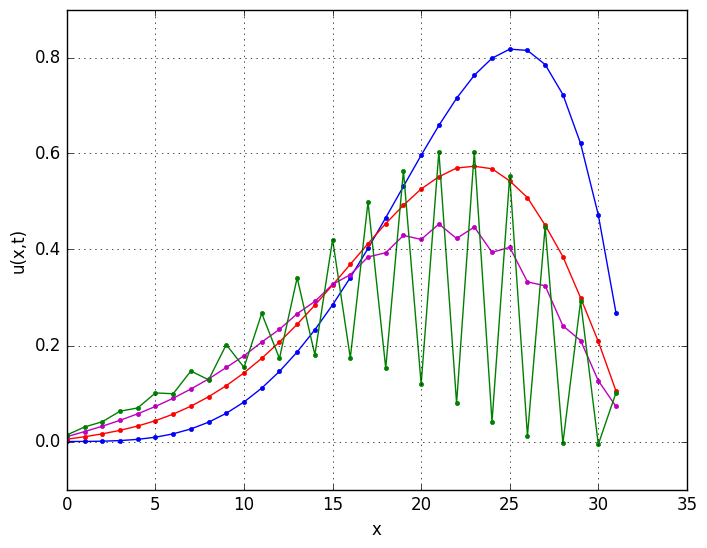In :
λ,V = eig(L)
plot(λ, "bo")
ylabel(L"\lambda")
xlabel("n")
grid("on")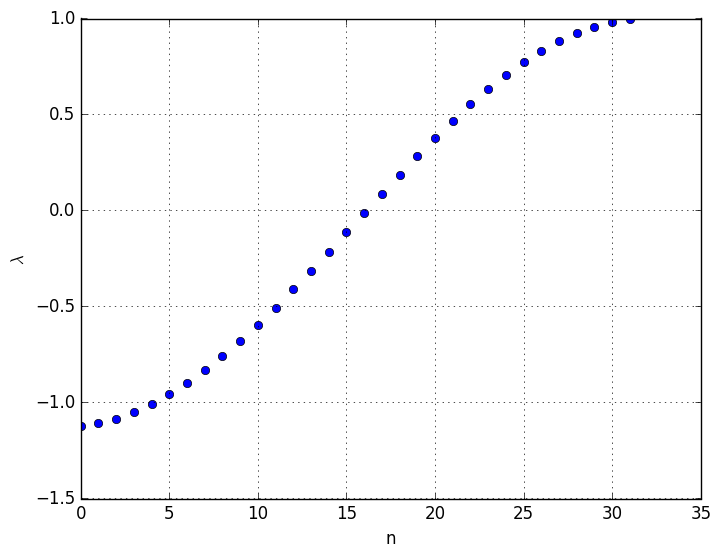In :
Lx = 1      # spatial domain is [0, Lx]
ν  = 1/8    # diffusion constant
T  = 1/2    # total integration time

Nx = 32    # number of gridpoints
Δt = 1/64  # time step

Nt = round(Int, T/Δt)  # total number of timesteps
Δx = Lx/(Nx+1)   # grid spacing
x = Δx*(1:Nx)    # interior gridpoints
t = Δt*(0:Nt-1)  # time steps

# Set up Crank-Nicolson time-stepping formula
# [I - ν Δt/(2Δx²) D₂] u^{n+1} = [I + ν Δt/(2Δx²) D₂] u^n
#                   A u^{n+1} = B u^n
D₂ = diagm(-2*ones(Nx)) + diagm(ones(Nx-1), -1) + diagm(ones(Nx-1),1)
A  = I - (ν * Δt/(2Δx^2)) * D₂
B  = I + (ν * Δt/(2Δx^2)) * D₂

U = zeros(Nx,Nt)
U[:,1] = 10*(x.^4).*(Lx-x)  # an initial condition that fits the BCs

for n = 2:Nt
U[:,n] = A\(B*U[:,n-1])
end

using PyPlot
pcolor(x,t,U',vmin=0, vmax=1)
colorbar()
xlabel("x")
ylabel("t")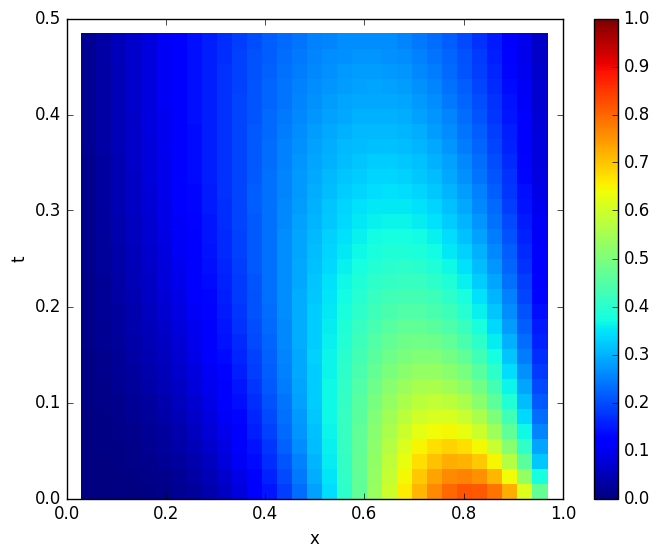Out:
PyObject <matplotlib.text.Text object at 0x7fab1a744950>
In :
λ,V = eig(B,A)
plot(λ, "bo")
ylabel(L"\lambda")
xlabel("n")
grid("on")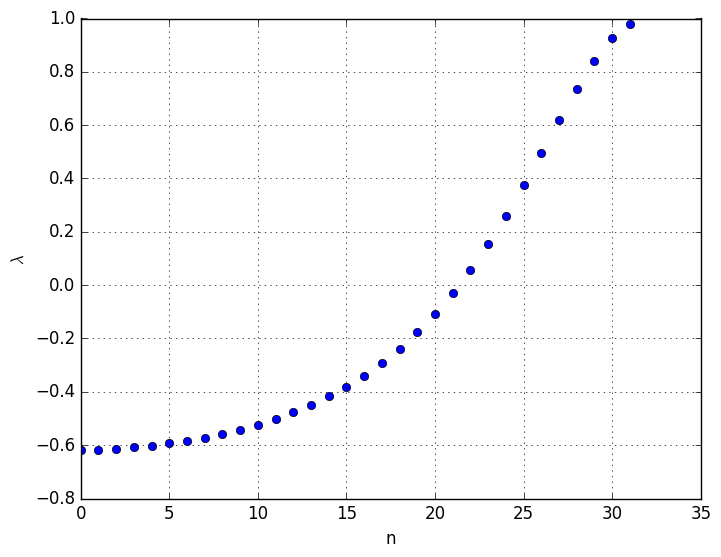In [ ]: